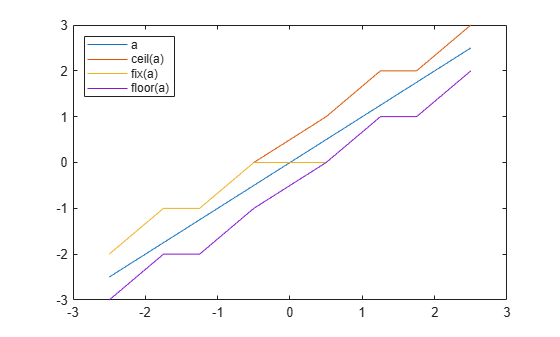# ceil

Rounds toward positive infinity

## Syntax

``y = ceil(a)``

## Description

example

````y = ceil(a)` rounds `fi` object `a` to the nearest integer in the direction of positive infinity and returns the result in `fi` object `y`.```

## Examples

collapse all

The following example demonstrates how the `ceil` function affects the `numerictype` properties of a signed `fi` object with a word length of 8 and a fraction length of 3.

`a = fi(pi,1,8,3)`
```a = 3.1250 DataTypeMode: Fixed-point: binary point scaling Signedness: Signed WordLength: 8 FractionLength: 3 ```
`y = ceil(a) `
```y = 4 DataTypeMode: Fixed-point: binary point scaling Signedness: Signed WordLength: 6 FractionLength: 0 ```

The following example demonstrates how the `ceil` function affects the `numerictype` properties of a signed `fi` object with a word length of 8 and a fraction length of 12.

`a = fi(0.025,1,8,12)`
```a = 0.0249 DataTypeMode: Fixed-point: binary point scaling Signedness: Signed WordLength: 8 FractionLength: 12 ```
`y = ceil(a)`
```y = 1 DataTypeMode: Fixed-point: binary point scaling Signedness: Signed WordLength: 2 FractionLength: 0 ```

The functions `ceil`, `fix`, and `floor` differ in the way they round `fi` objects:

• The `ceil` function rounds values to the nearest integer toward positive infinity.

• The `fix` function rounds values to the nearest integer toward zero.

• The `floor` function rounds values to the nearest integer toward negative infinity.

This example illustrates these differences for a given `fi` input object `a`.

```a = fi([-2.5,-1.75,-1.25,-0.5,0.5,1.25,1.75,2.5]'); y = [a ceil(a) fix(a) floor(a)]```
```y=8×4 object -2.5000 -2.0000 -2.0000 -3.0000 -1.7500 -1.0000 -1.0000 -2.0000 -1.2500 -1.0000 -1.0000 -2.0000 -0.5000 0 0 -1.0000 0.5000 1.0000 0 0 1.2500 2.0000 1.0000 1.0000 1.7500 2.0000 1.0000 1.0000 2.5000 3.0000 2.0000 2.0000 DataTypeMode: Fixed-point: binary point scaling Signedness: Signed WordLength: 16 FractionLength: 13 ```
`plot(a,y); legend('a','ceil(a)','fix(a)','floor(a)','location','NW');`## Input Arguments

collapse all

Input `fi` array, specified as scalar, vector, matrix, or multidimensional array.

For complex `fi` objects, the imaginary and real parts are rounded independently.

`ceil` does not support `fi` objects with nontrivial slope and bias scaling. Slope and bias scaling is trivial when the slope is an integer power of 2 and the bias is 0.

Data Types: `fi`
Complex Number Support: Yes

## Algorithms

• `y` and `a` have the same `fimath` object and `DataType` property.

• When the `DataType` property of `a` is `single`, `double`, or `boolean`, the `numerictype` of `y` is the same as that of `a`.

• When the fraction length of `a` is zero or negative, `a` is already an integer, and the `numerictype` of `y` is the same as that of `a`.

• When the fraction length of `a` is positive, the fraction length of `y` is `0`, its sign is the same as that of `a`, and its word length is the difference between the word length and the fraction length of `a`, plus one bit. If `a` is signed, then the minimum word length of `y` is `2`. If `a` is unsigned, then the minimum word length of `y` is `1`.

## Extended Capabilities

### HDL Code GenerationGenerate Verilog and VHDL code for FPGA and ASIC designs using HDL Coder™.